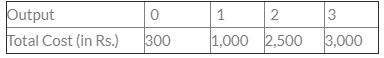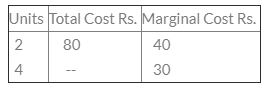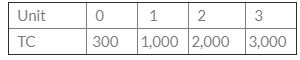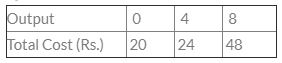Courses

# Test: Theory Of Cost- 2

## 30 Questions MCQ Test Business Economics for CA Foundation | Test: Theory Of Cost- 2

Description
This mock test of Test: Theory Of Cost- 2 for CA Foundation helps you for every CA Foundation entrance exam. This contains 30 Multiple Choice Questions for CA Foundation Test: Theory Of Cost- 2 (mcq) to study with solutions a complete question bank. The solved questions answers in this Test: Theory Of Cost- 2 quiz give you a good mix of easy questions and tough questions. CA Foundation students definitely take this Test: Theory Of Cost- 2 exercise for a better result in the exam. You can find other Test: Theory Of Cost- 2 extra questions, long questions & short questions for CA Foundation on EduRev as well by searching above.
QUESTION: 1

Solution:
QUESTION: 2

Solution:
QUESTION: 3

### At which point does the marginal cost curve intersect the average variable cost curve and short run average total cost curve?

Solution:
QUESTION: 4

What is the total cost of production of 20 units, if fixed cost is Rs. 5,000 and variable cost is Rs. 2/-?

Solution:
QUESTION: 5

A firm’s average fixed cost is Rs. 40 at 12 units. What will be the average fixed cost at 8 units:

Solution:
QUESTION: 6

AFC curve is:

Solution:
QUESTION: 7

A firm producing 7 units of output has an average total cost of Rs. 150 and has to pay Rs. 350 to its fixed factors of production. How much of the average total cost is made up of variable cost ?

Solution:
QUESTION: 8

The Average fixed cost for producing on output of 6 units of a product by a firm is Rs. 30. The same cost for producing an output of 4 units will be Rs. ______.

Solution:
QUESTION: 9

Which of the following cost curves is never ‘U’ shaped?

Solution:
QUESTION: 10

Marginal cost changes due to change in ________ cost.

Solution:
QUESTION: 11

Average total cost to a firm is Rs. 600 when it produces 10 units of output and Rs 640 when the output is 11 units. The MC of the 11th unit is:

Solution:
QUESTION: 12

Which of the following curves never touch any axis but is downward?

Solution:
QUESTION: 13

U-shaped average cost curve is based on:

Solution:
QUESTION: 14

Direct costs are also known as _______.

Solution:
QUESTION: 15

Suppose the total cost production of a commodity ‘x’ is Rs. 1, 25,000 out of which Implicit cost is Rs. 35,000 and normal profit is Rs. 25,000. What would be the explicit cost of commodity x?

Solution:

125000-25000-35000 = 65000 = explicit cost.
Total cost = implicit cost + explicit cost.

QUESTION: 16

What will be the AFC of 3 units of Output as per table given below?Solution:
QUESTION: 17

Find AFC of 3 units:
Unit            0   1    2   3
Total cost 15  25  35  45

Solution:
QUESTION: 18

Calculate total cost of 4 units:Solution:
QUESTION: 19

The total cost of production of 10 units is Rs. 200. When production is increased to 20 units its total cost becomes Rs. 600. What will be its marginal cost.

Solution:
QUESTION: 20

From the following details, firm out the average variable cost of 10 units:
OUTPUT :    0                 10        20
Total cost : Rs. 200 Rs. 400 Rs. 800

Solution:
QUESTION: 21

Long run does not have:

Solution:
QUESTION: 22

Which one of the following is correct?

Solution:
QUESTION: 23

Find out AFC of 3 unit:Solution:
QUESTION: 24

In the long run all factors are-----

Solution:
QUESTION: 25

Average Revenue Curve is also known as _________.

Solution:
QUESTION: 26

A forms AFC is Rs. 200 at 10 units of output what will be it at 20 units of output?

Solution:
QUESTION: 27

Which of the following is known as Envelop Curve?

Solution:
QUESTION: 28

GivenWhat will be the AFC of 4 units of Output

Solution:

QUESTION: 29

Payment made to outsiders for their goods and services are called :

Solution:
QUESTION: 30

Labour is hirable but you cannot hire:

Solution: